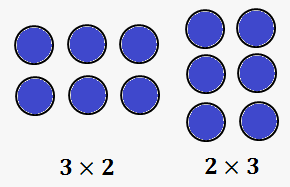# Commutative Property of Multiplication

Commutative property of multiplication:

$\color{purple}{a\times b=b\times a}$What does it mean?

It means, that order of numbers doesn't matter.

Indeed, as can be seen from illustration, we can count there are 3 circles in a row, and there are 2 rows, so total number of squares is ${3}\times{2}={6}$.

From another side, we can rotate picture and count circles in another way: 3 rows, and in each row 2 circles: ${2}\times{3}={6}$.

Warning: it doesn't work with division, i.e. $\frac{{a}}{{b}}\ne\frac{{b}}{{a}}$.

For example, $\frac{{3}}{{2}}\ne\frac{{2}}{{3}}$.

However, commutative property of multiplication works for negative numbers (in fact, for real numbers) as well.

Example 1. ${3}\times{\left(-{4}\right)}={\left(-{4}\right)}\times{3}=-{12}$.

Example 2. ${\left(-{2.51}\right)}\times{\left(-{3.4}\right)}={\left(-{3.4}\right)}\times{\left(-{2.51}\right)}$.

Example 3. $-\frac{{5}}{{8}}\times\frac{{2}}{{3}}={\left(-\frac{{5}}{{8}}\right)}\times\frac{{2}}{{3}}=-\frac{{5}}{{12}}$.

Conclusion. So, the basic rule here is following: whenever you see multiplication, you can interchange factors.